Abstract: 本文以线性变换的角度重新理解矩阵变换的原理，以对角化和SVD作为主要的案例
Keywords: Diagonalization，Pseudoinverse

# 对角化和伪逆

## 对角化和伪逆(Diagonalization and the Pseudoinverse)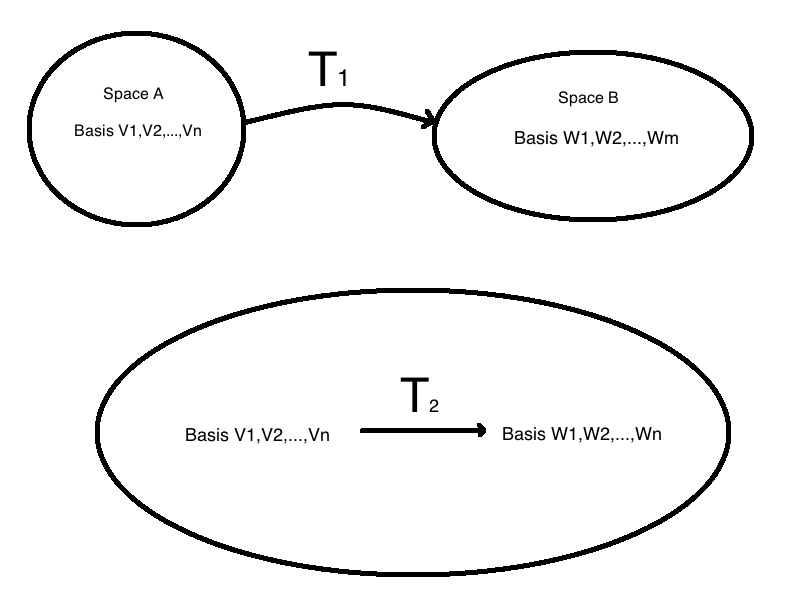$$w=A\vec{v}$$

$$w’=Bw=BA\vec{v}$$

$$w’=Bw=BA\vec{v}=C\vec{v}\\ C\vec{v}=BA\vec{v}$$

$$CI\dot{v}=BAI\dot{v}\\ CI=BAI$$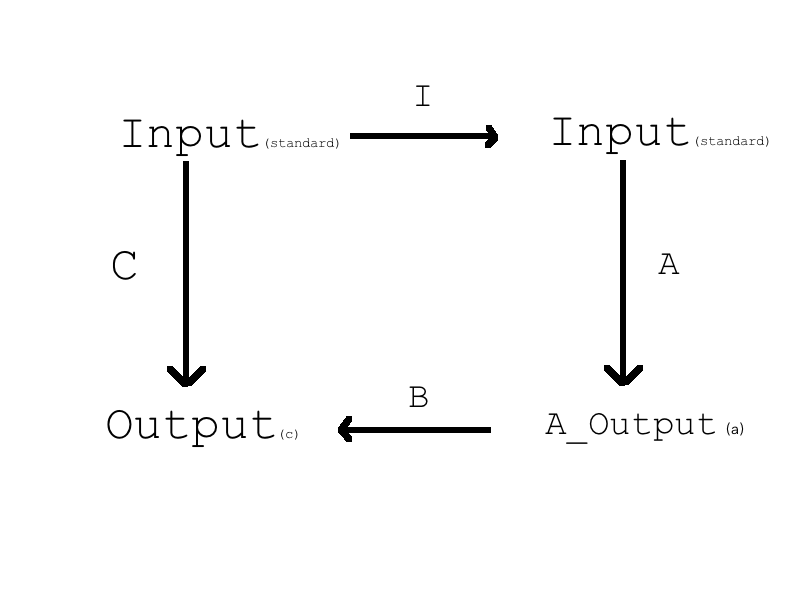## 相似矩阵 :$A$ and $S^{-1}AS$ and $W^{-1}AW$

$$B=M^{-1}AM\\$$

eg. Project onto the line $y=-x$ that goes from northwest to southeast. The vector $(1,0)$ projects to $(.5,.5)$ ,the projection of $(0,1)$ is $(-.5,.5)$

1. 按照标准做法，选取标准基，我们可以得到线性变换矩阵 $A=\begin{bmatrix}.5&-.5\newline -.5&.5\end{bmatrix}$ 。
2. 如果我们想要对角矩阵，需要先求出特征向量矩阵 $S=\begin{bmatrix}1&1\newline-1&1\end{bmatrix}$ 对应的求出在 $S^{-1}$ 基下的线性变换矩阵 $\Lambda=\begin{bmatrix}1&0\\0&0\end{bmatrix}$ 果然很漂亮然后把输出再变换到$S$ 为基的形式就回到了上面的A
3. 求相似和2类似这里不再赘述

## 奇异值分解(SVD)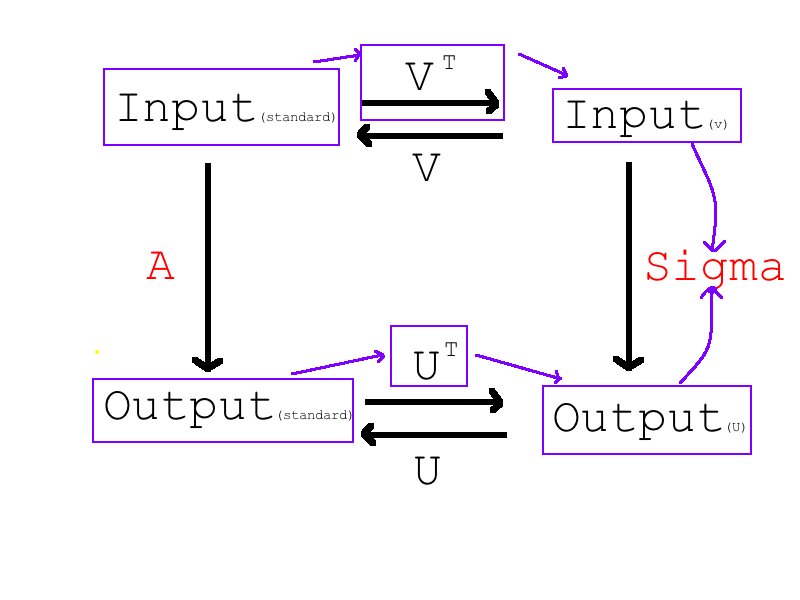## 伪逆(The Pseudoinverse)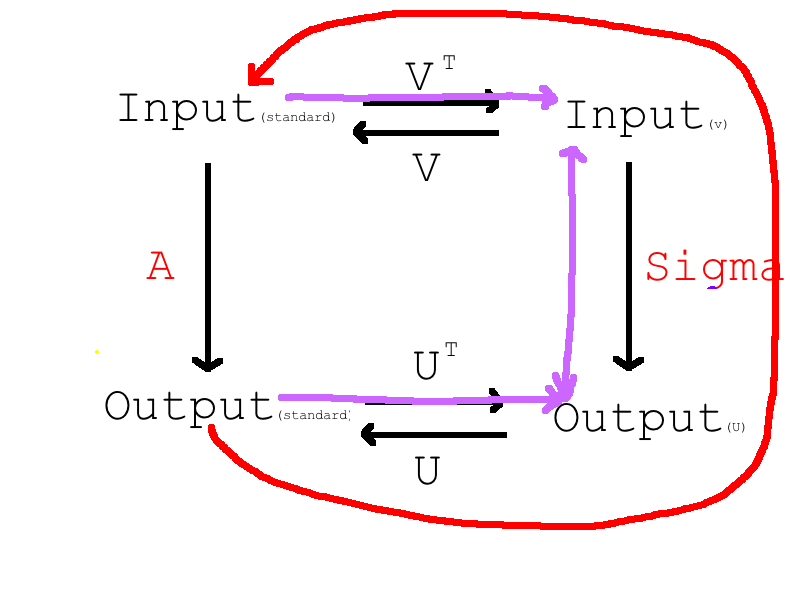$$\Sigma_r^{-1}= \begin{bmatrix} \sigma_1^{-1}&&\\ &\ddots&\\ &&\sigma_r^{-1} \end{bmatrix}\\ \Sigma^{+}= \begin{bmatrix} \sigma_1^{-1}&&&&&\\ &\ddots&&&&\\ &&\sigma_r^{-1}&&&\\ &&&0&&\\ &&&&\ddots&\\ &&&&&0 \end{bmatrix}$$

$$\Sigma^{+}\Sigma = \begin{bmatrix} 1&&&&&\\ &\ddots&&&&\\ &&1&&&\\ &&&0&&\\ &&&&\ddots&\\ &&&&&0 \end{bmatrix}$$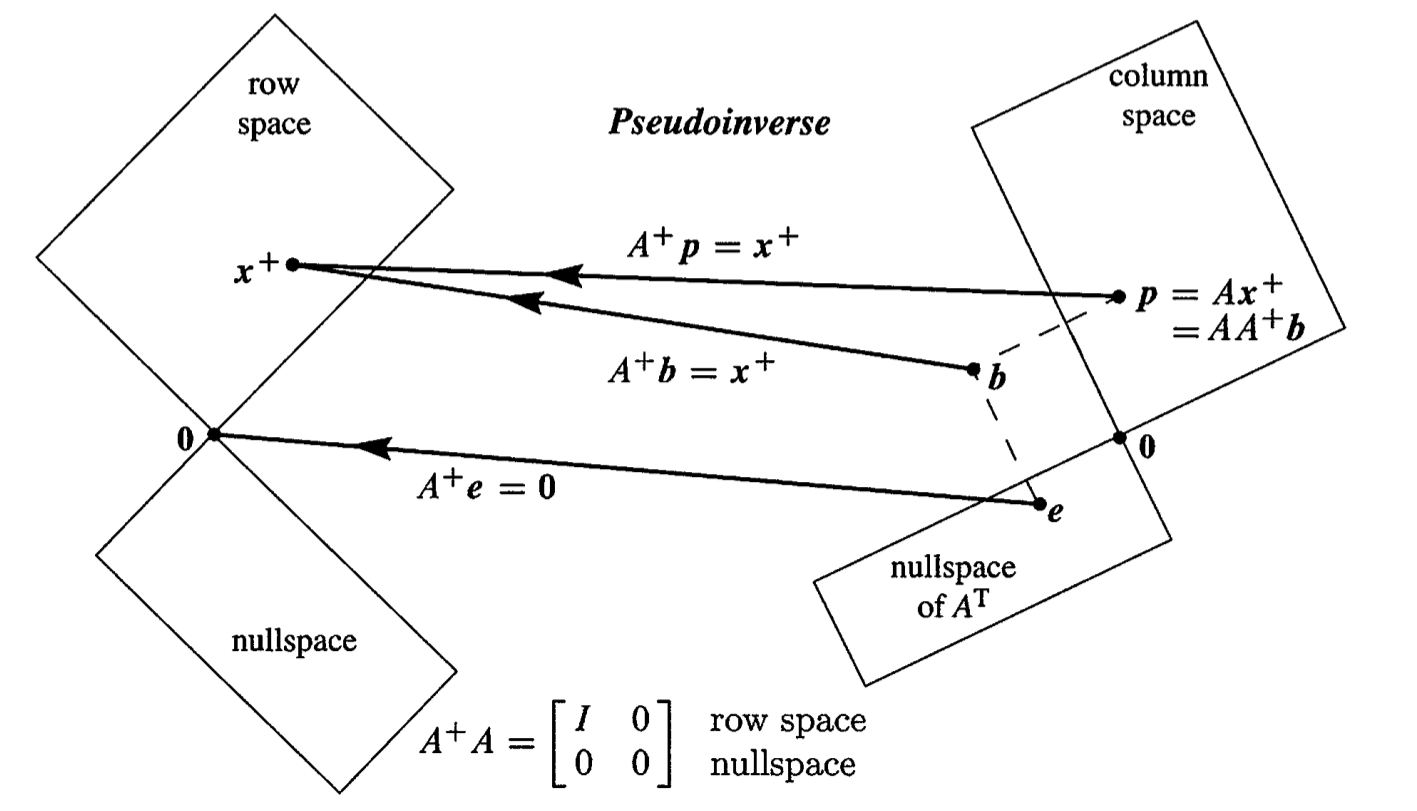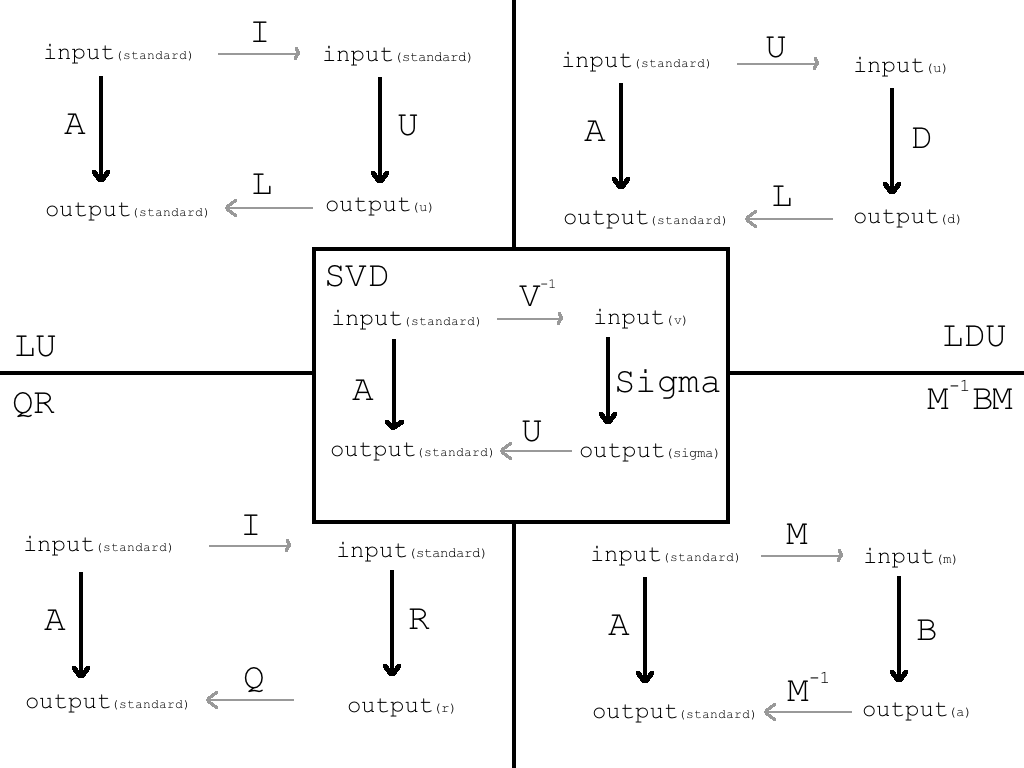0%